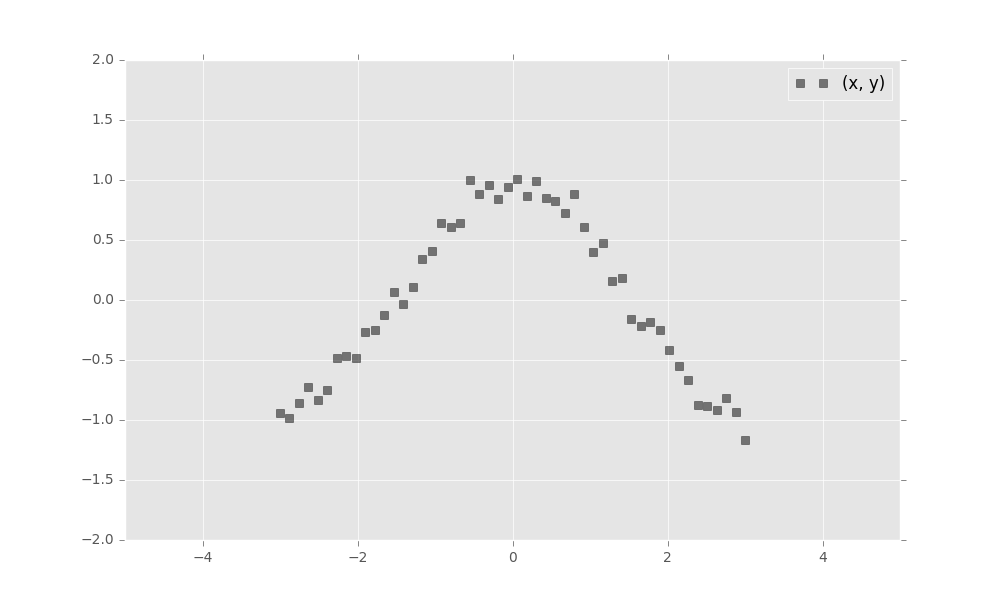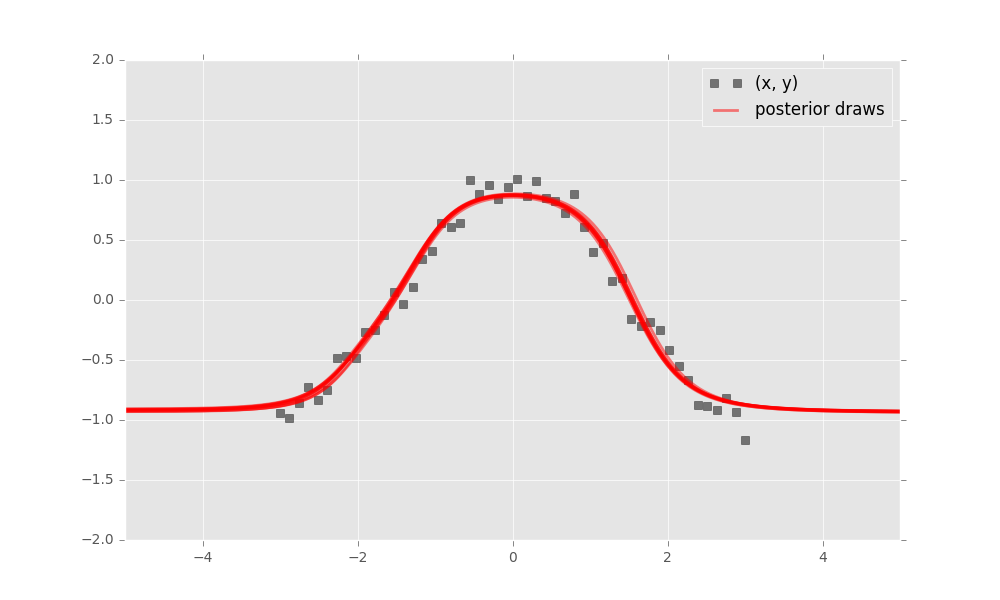## Getting Started

Getting started with Edward is easy.

### Installation

To install the latest stable version, run

pip install edward

To install the latest development version, run

pip install -e "git+https://github.com/blei-lab/edward.git#egg=edward"

See the troubleshooting page for Edward’s dependencies and more details.

Probabilistic modeling in Edward uses a simple language of random variables. Here we will show a Bayesian neural network. It is a neural network with a prior distribution on its weights. (This example is abridged; an interactive version with Jupyter notebook is available here.)

First, simulate a toy dataset of 50 observations with a cosine relationship.

import numpy as np

x_train = np.linspace(-3, 3, num=50)
y_train = np.cos(x_train) + np.random.normal(0, 0.1, size=50)
x_train = x_train.astype(np.float32).reshape((50, 1))
y_train = y_train.astype(np.float32).reshape((50, 1))Next, define a two-layer Bayesian neural network. Here, we define the neural network manually with tanh nonlinearities.

import tensorflow as tf
from edward.models import Normal

W_0 = Normal(loc=tf.zeros([1, 2]), scale=tf.ones([1, 2]))
W_1 = Normal(loc=tf.zeros([2, 1]), scale=tf.ones([2, 1]))
b_0 = Normal(loc=tf.zeros(2), scale=tf.ones(2))
b_1 = Normal(loc=tf.zeros(1), scale=tf.ones(1))

x = x_train
y = Normal(loc=tf.matmul(tf.tanh(tf.matmul(x, W_0) + b_0), W_1) + b_1,
scale=0.1)

Next, make inferences about the model from data. We will use variational inference. Specify a normal approximation over the weights and biases.

qW_0 = Normal(loc=tf.get_variable("qW_0/loc", [1, 2]),
scale=tf.nn.softplus(tf.get_variable("qW_0/scale", [1, 2])))
qW_1 = Normal(loc=tf.get_variable("qW_1/loc", [2, 1]),
scale=tf.nn.softplus(tf.get_variable("qW_1/scale", [2, 1])))
qb_0 = Normal(loc=tf.get_variable("qb_0/loc", ),
scale=tf.nn.softplus(tf.get_variable("qb_0/scale", )))
qb_1 = Normal(loc=tf.get_variable("qb_1/loc", ),
scale=tf.nn.softplus(tf.get_variable("qb_1/scale", )))

Defining tf.get_variable allows the variational factors’ parameters to vary. They are all initialized at 0. The standard deviation parameters are constrained to be greater than zero according to a softplus transformation.

Now, run variational inference with the Kullback-Leibler divergence in order to infer the model’s latent variables given data. We specify 1000 iterations.

import edward as ed

inference = ed.KLqp({W_0: qW_0, b_0: qb_0,
W_1: qW_1, b_1: qb_1}, data={y: y_train})
inference.run(n_iter=1000)

Finally, criticize the model fit. Bayesian neural networks define a distribution over neural networks, so we can perform a graphical check. Draw neural networks from the inferred model and visualize how well it fits the data.The model has captured the cosine relationship between $$x$$ and $$y$$ in the observed domain.